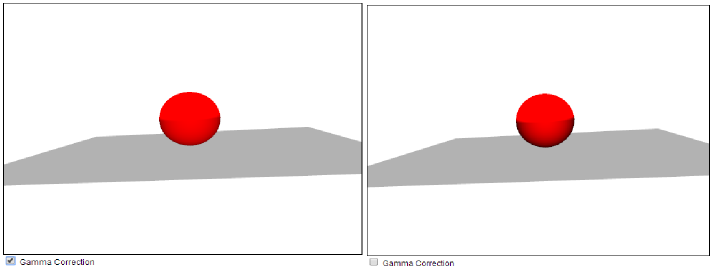## Gamma Correction

### Short facts

If you know what gamma correction is, you may just want to know that: Lighting in X3DOM can be gamma-correct or not, depending on the Environment node’s gammaCorrectionDefault field. RGB Colors and textures are assumed to be gamma coded. Intensities of all sorts are assumed to be linear. You can use gamma correction with the "gammaCorrectionDefault" attribute:

``````
<Environment id="gamma" gammaCorrectionDefault="linear"></Environment>
``````
Even with a simple sphere we can see the difference:gamma correction in action

### Slightly longer version

X3DOM lighting works based on X3D’s lighting equations. These are defined in the X3D standard document. However, there is some debate on how such equations might be implemented. Some say they must be implemented by interpreting the word “intensity” in the X3D spec to mean precisely luminous intensity whereas others say it’s not worth the fuss to deal with such peculiarities, so a straigt-forward implementation will suffice. X3D is not explicit about the issue, so everyone generally does as he pleases.

X3DOM offers you both options, so you can work the way you want. In the non-interactive rendering community, this is better known as having a “linear workflow” or not. These days people generally prefer the linear workflow, i.e. compensating for gamma-coded colors in some way, or eliminating them from the scene and assets.

## Is this about tone mapping?

Tone mapping uses the similar means but has a different objective. Gamma correction is simply about getting render calculations right in the first place, while tone mapping is about presenting renderings to the eye in a pleasant way.
Tone mapping is not yet implemented in X3DOM.

## How does it work?

X3DOM has a field in the Environment bindable to control how lighting equations are being implemented, the gammaCorrectionDefault field. This field therefore controls whether your lighting is to be calculated linear (gamma being compensated) or not (retaining gamma in all calculations).

The field may have one of three states:

• “linear” (default) The preferred gamma coefficient (2.2, close to the sRGB standard’s and Rec. 709 transfer function) is applied. Sometimes called “gamma correct[ed]”.
• “fastLinear” A faster (in terms of GPU cycles), albeit slightly off transfer function based on sqrt. Equivalent to a gamma of 2.0.
• “none” Do not correct for gamma coding, assume a gamma of 1.0. Confusingly, this results in a linear (identity) degenerate gamma correction which causes results to be processes as (non-linear as) they are stored.

All textures and RGB colors are assumed to be gamma coded (the norm for 99.9% of image material). Inherently linear values like intensity of lights, shadow intensity or shininess are assumed to be linear. The setting just controls how these assumptions are being implemented. This means that generally there is no need for big changes (like linearizing all colors) when switching to (or away from) gamma-correct lighting. Note that while the setting affects how lighting information in the scene is being treated, the more important part is how it affects all the intermediaries in the lighting calculations that needs to be performed in other to light up your scene. The “moon gamma” example shows how lighting is affected by gamma. Generally, gamma-correct renderings tend to be brighter because in the majority of cases, not accouting for gamma loses light energy. This is especially true in the mid tones which are important to many scenes. In principle the worst case is about 2.5 times less light than a corrected implementation, but under circumstances this bias can accumulate.

## Linear lighting in practice

It is worth mentioning that the human visual system has a non-linear lightness perception. Gamma is, to some extent, the video/computer graphics response to this fact.

When working gamma-corrected (i.e. in the linear setting), intensities are treated as optically linear. Thus, when working with them it is best to adapt the techniques that work in optics. One that is easy to adapt is the concept of stops, which denotes 2-times increases or decreases in exposure, i.e. in the amount of light available to capture a photo:
+n stops = intensity * (2 ^ n)
That is, an intensity difference known as “one stop” can be achieved by halving or doubling the intensity. A human generally perceives 1/5th (more precisely, 18.1%) of a given intensity as “half as bright”. So a stop less is half as much light (in the sense of energy), but is not perceived to be half as bright.

It is possible, but quite awkward, to come up with equivalent equations for uncorrected lighting (the none setting).